Privacy-Preserving Computer Vision with Homomorphic Encryption

Stanley Black and Decker Seminar

June 3, 2021

## Key Driver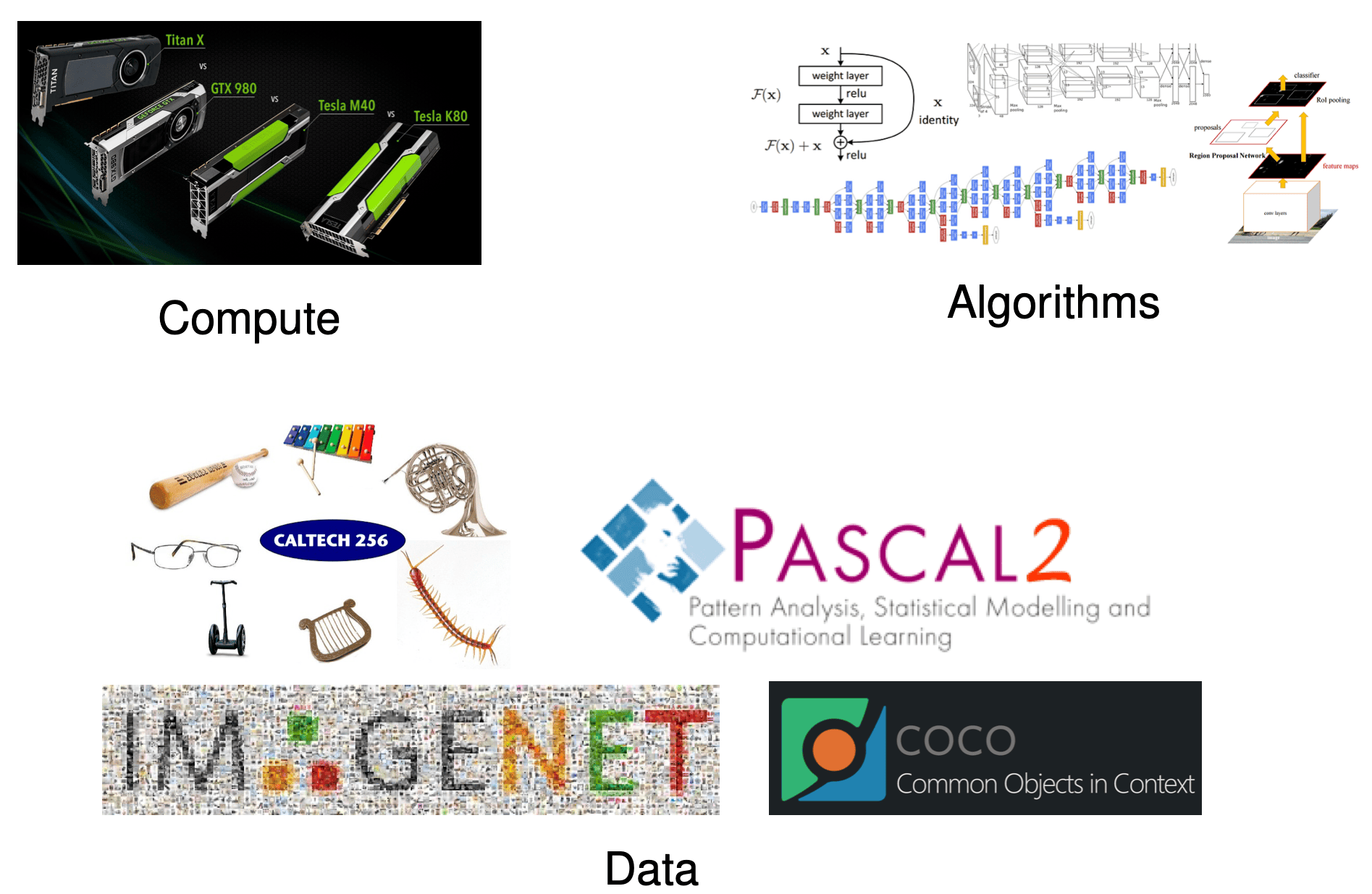## Dark Data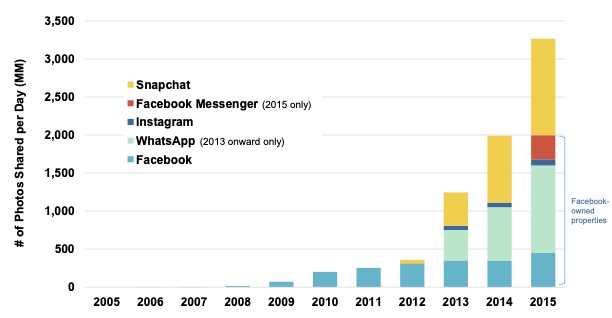• About 3.5 billion photos are shared publicly.
• Conservatively, $2\times$-$3\times$ are not shared publicly.
• Sensitive data: medical images, surveillance images.
• Private learning:
• enables free and open sharing
• build better computer vision models

## Why Privacy in Computer Vision...consent should be given for all purposes...
"The Secretive Company That Might End Privacy as We Know It"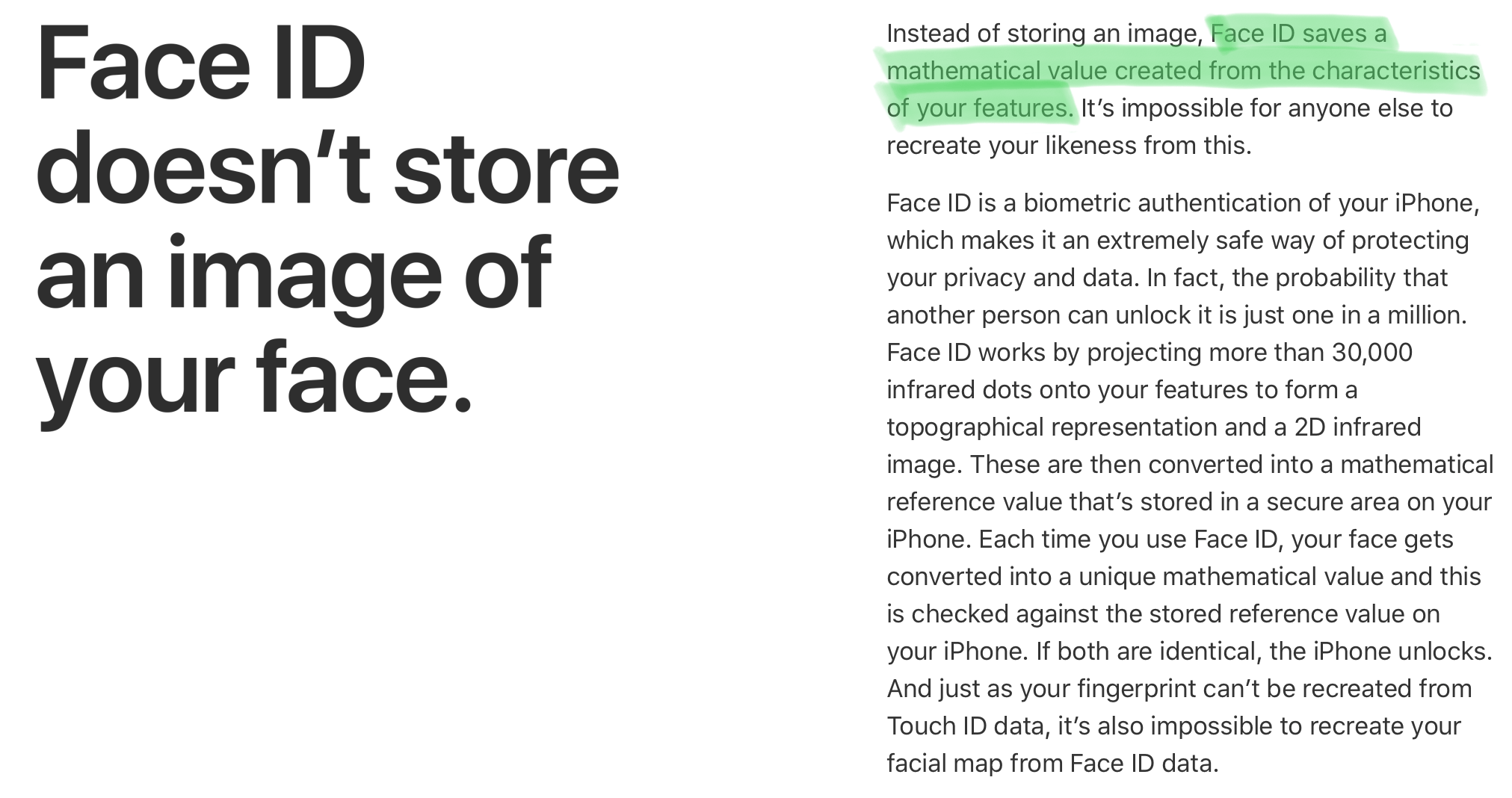Privacy Preserving Computer Vision

Mechanism: machine learning on encrypted data

## Encryption: The Holy Grail?

• Data encryption is an attractive option
• protects user's privacy
• enables free and open sharing
• mitigate legal and ethical issues

• Encryption scheme needs to allow computations directly on the encrypted data.
• Solution: Homomorphic Encrpytion

## What is Homomorphic Encryption?

• Encryption that allows computations on ciphertext.
• Partially Homomorphic Encryption: allows homomorphic additions or multiplications
• Somewhat Homomorphic Encryption: allows limited number of homomorphic additions and multiplications
• Fully Homomorphic Encryption: additions and multiplications

## Key Idea of Homomorphic Encryption

Ring Learning with Errorsop plaintext ciphertext
$x$ $(x + e_1) \mbox{ mod } t$
$y$ $(y + e_2) \mbox{ mod } t$
$+$ $x+y$ $(x+y + e_3') \mbox{ mod } t$
$\times$ $x\times y$ $(x\times y + e_4'') \mbox{ mod } t$

## Machine Learning and Homomorphic Encryption

• Main Challenges:
• Homomorphic Encryption:
• Supports only basic arithmetic operations (additions, subtractions, multiplications and divisions)
• Each basic operation is computationally expensive.
• Machine Learning:
• Requires complex mathematical operations, including non-linear operations.
• Computationally challenging in it's own right (big-data, large models).

## Today's Agenda

• Leverage the advantages of ML and HE while overcoming their challenges.
• Redesign ML algorithms with constraints of HE in mind.
• Privacy-Preserving Visual Learning Using Doubly Permuted Homomorphic Encryption, ICCV 2017
• Redesign HE algorithms with constraints of ML in mind.
• Secure Face Matching Using Fully Homomorphic Encryption, BTAS 2018
• HERS: Homomorphically Encrypted Representation Search, Arxiv 2020

## Learning from Private Data: Federated Learning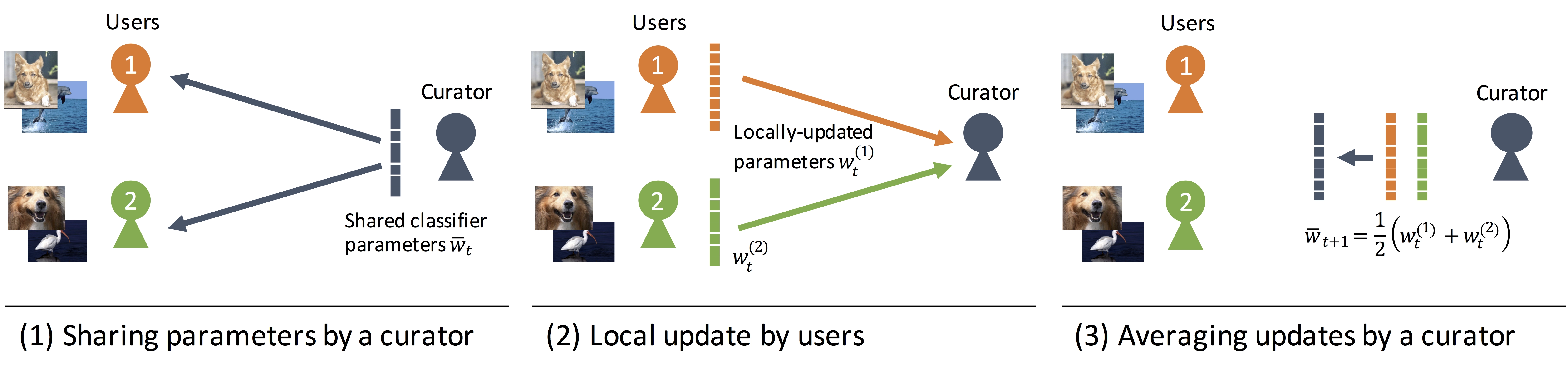• Distributed learning of parameters from private data.
• Clients download current global model $\bar{\mathbf{w}_t}$.
• Client updates model from local data.
• Yonetani et. al., "Privacy-Preserving Visual Learning Using Doubly Permuted Homomorphic Encryption", ICCV 2017

## Private Learning: Differentially Private SGD• Differential Privacy [Dwork et.al. 2006]
• Limitation: Trade-off accuracy for privacy

## Private Learning: Homomorphic Encryption

• Paillier Encryption: only allows addition of integers
• Encryption Key $m$
• Base $g$
• random $r \in \{0,\dots,m-1\}$
• Ciphertext of $x$: $\xi(x) = g^xr^m \mod m^2$
• Addition, $x_1 + x_2$, in the encrypted domain. \begin{eqnarray} \xi(x_1)\xi(x_2) &=& (g^{x_1}r_1^m)(g^{x_2}r_2^m)\mod m^2 \nonumber \\ &=& g^{x_1+x_2}(r_1r_2)^m \mod m^2 \nonumber \\ &=& \xi(x_1+x_2) \nonumber \end{eqnarray}

## Encrypted Federated Learning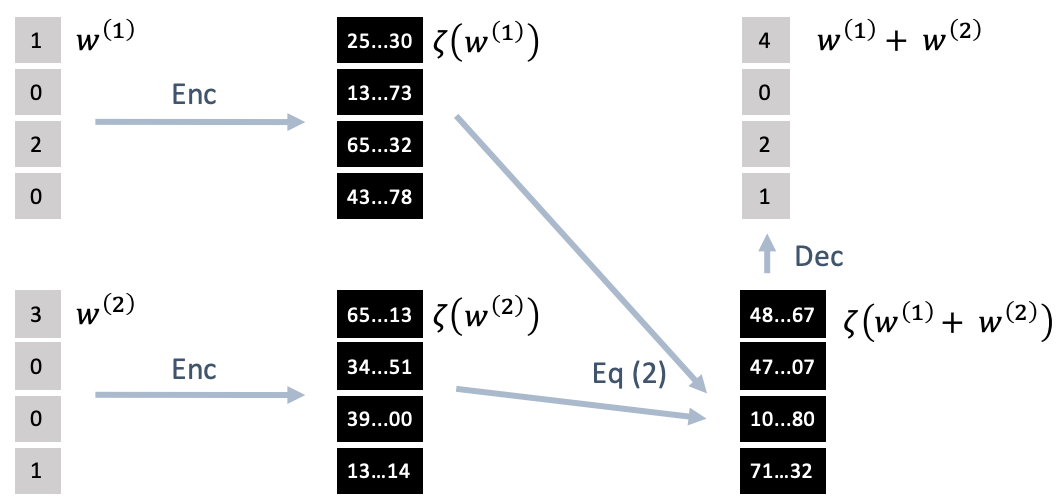• Yonetani et. al., "Privacy-Preserving Visual Learning Using Doubly Permuted Homomorphic Encryption", ICCV 2017

## Homomorphic Encryption for Sparse Data

• Sparse Data $\mathbf{x} \in \mathbb{R}^d$: $\mathbf{x} = \mathbf{K}\mathbf{v}$
• $m$: number of non-zero elements
• $\mathbf{v} \in \mathbb{R}^m$: real non-zero values
• $\mathbf{K} \in \{0,1\}^{d \times m}$: binary non-zero support matrix
• Doubly-Permuted Homomorphic Encryption for Sparse Data:
• Choose encryption capacity $m \leq n \leq d$
• Encrypt $\mathbf{v}_i \in \mathbb{R}^n$: $\xi(\mathbf{v}_i)$
• Apply global permutation $\phi$ on $\mathbf{K}_i \in \{0,1\}^{d \times n}$: $\mathbf{K}'=\phi\mathbf{K}_i$
• Apply local permutation $\phi_i$ on $\mathbf{K}_i' \in \{0,1\}^{d \times n}$: $\mathbf{K}_i''=\phi_i\mathbf{K}_i'$
• Yonetani et. al., "Privacy-Preserving Visual Learning Using Doubly Permuted Homomorphic Encryption", ICCV 2017

## Doubly-Permuted Homomorphic Encryption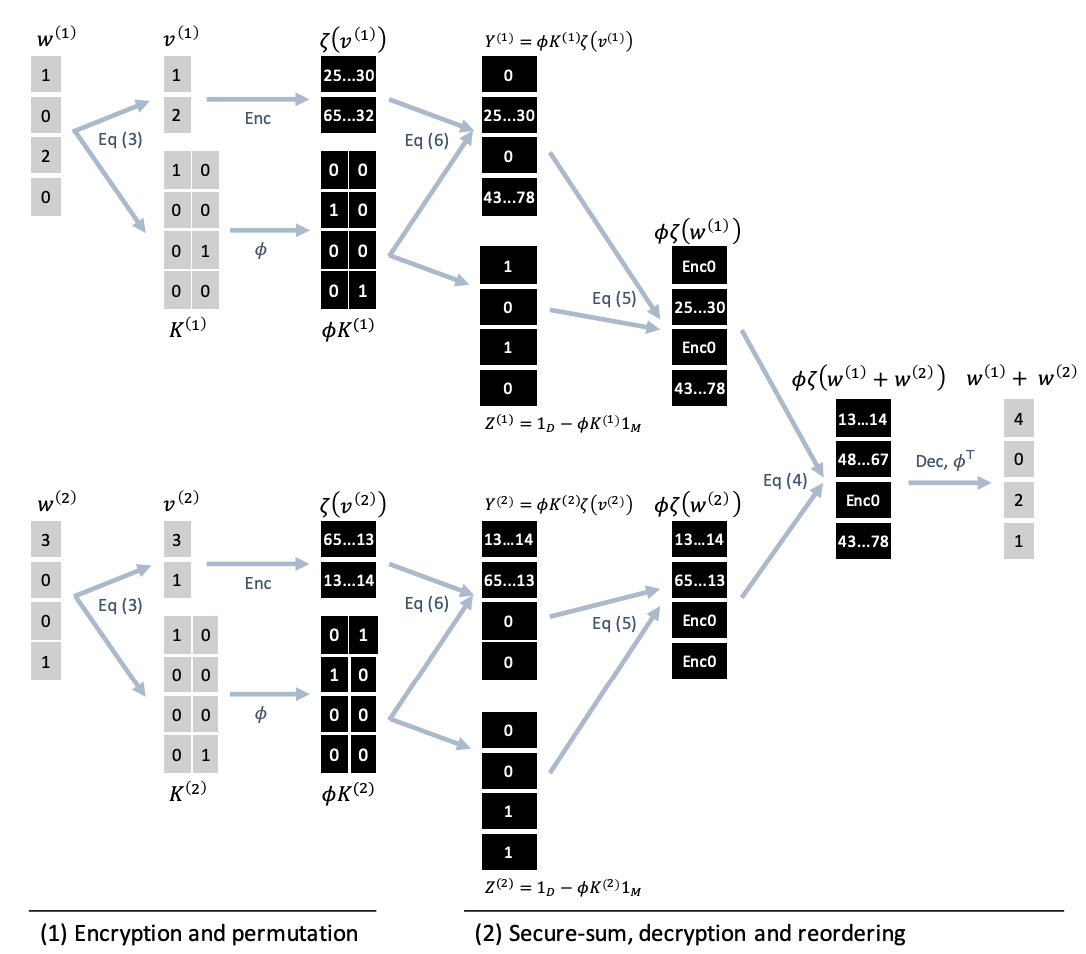• Yonetani et. al., "Privacy-Preserving Visual Learning Using Doubly Permuted Homomorphic Encryption", ICCV 2017

## Experiments: Real-World Evaluation

Facial Attribute RecognitionMethods Accuracy Privacy
LLWT15 87 No
DP 78 Yes
DP+SGD 64 Yes
Our Approach 84 Yes
• Yonetani et. al., "Privacy-Preserving Visual Learning Using Doubly Permuted Homomorphic Encryption", ICCV 2017

## Privacy Leakage in Augmented Reality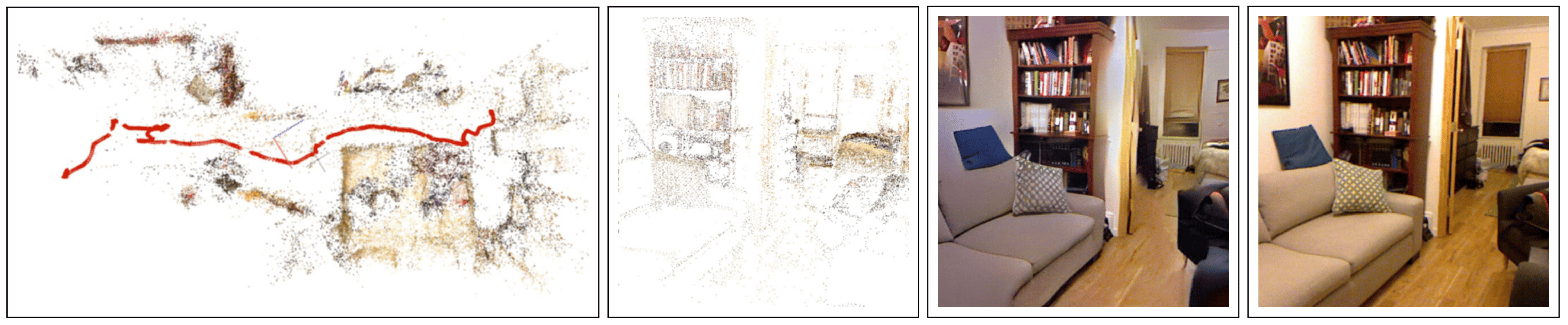• Pittaluga et. al., "Revealing Scenes by Inverting Structure from Motion Reconstructions", CVPR 2019

## Information Leakage from Representations

• Learned Embeddings:• Attacks on Embeddings:
• Face reconstruction from template
• Mai et. al., "On the reconstruction of face images from deep face templates," IEEE Transactions on Pattern Analysis and Machine Intelligence, 2018

## Secure Nearest Neigbhor Search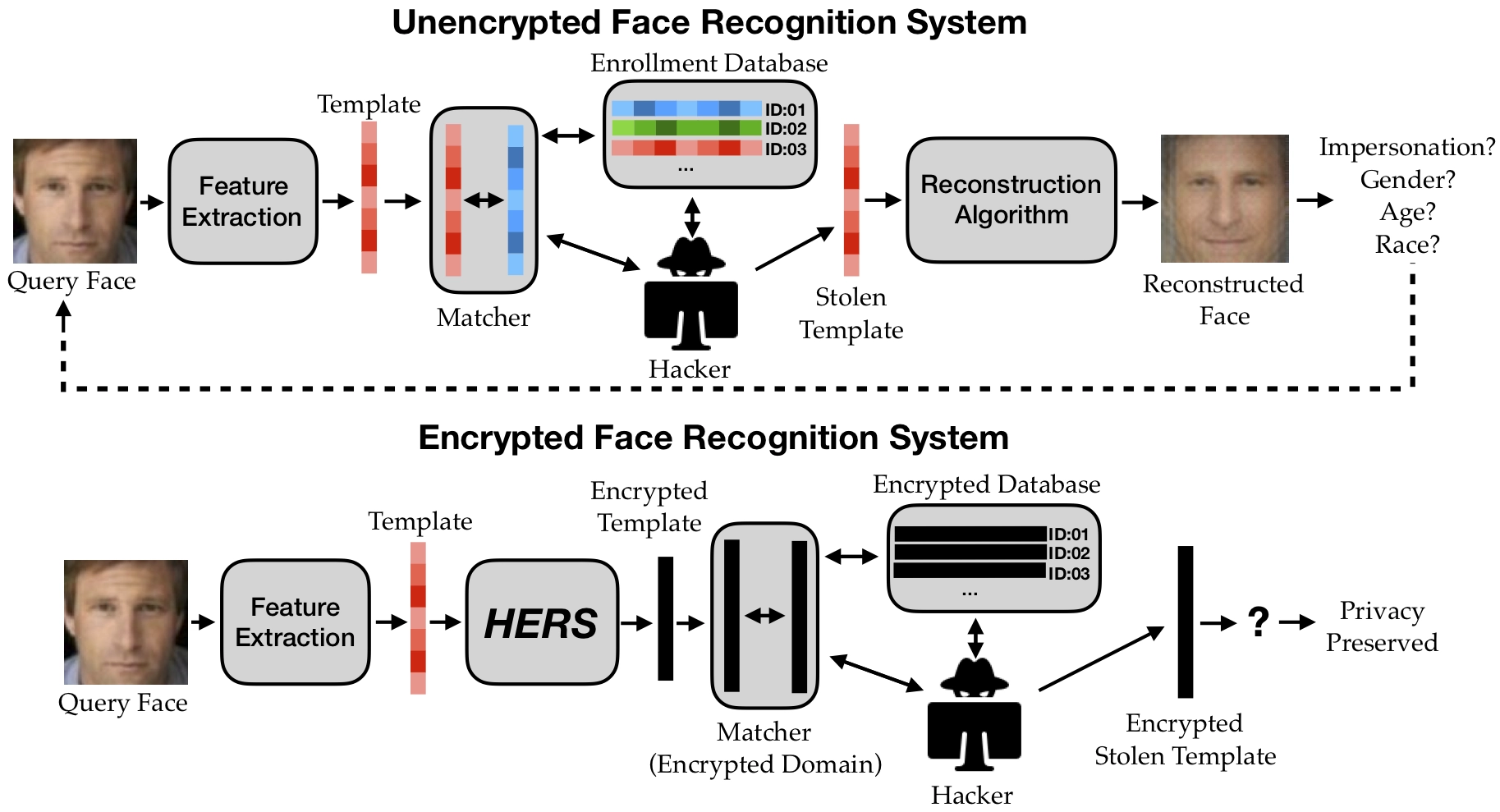• Englesma, Jain, Boddeti, "HERS: Homomorphically Encrypted Representation Search,", Arxiv 2020

## Enrollment Protocol

• Client Device:
• generates cryptographic keys
• captures image + extracts feature
• encrypts feature
• transmits encrypted feature + identity label to remote database• V.N. Boddeti, "Secure Face Matching Using Fully Homomorphic Encryption,", BTAS 2018

## Authentication Protocol

• Client Device:
• captures biometric signature + extracts feature, encrypts feature, transmits encrypted feature + claimed identity label to remote database
• Remote Database:
• homomorphic inner product between encrypted probe and gallery, transmits encrypted scores to client
• Client Device:
• decrypts received scores and makes decision• V.N. Boddeti, "Secure Face Matching Using Fully Homomorphic Encryption,", BTAS 2018

## Homomorphic Inner Products

• Feature Matching: \begin{eqnarray} \mbox{Euclidean Distance: } d(\mathbf{x},\mathbf{y}) &=& \|\mathbf{x}-\mathbf{y}\|^2_2 = \mathbf{x}^T\mathbf{x} + \mathbf{y}^T\mathbf{y} - 2\mathbf{x}^T\mathbf{y} \nonumber \\ \mbox{Cosine Similarity: } s(\mathbf{x},\mathbf{y}) &=& \frac{\mathbf{x}^T\mathbf{y}}{\|\mathbf{x}\|\|\mathbf{y}\|} \nonumber \end{eqnarray}
• Inner Product: $\mathbf{x}^T\mathbf{y}=\sum_{i=1}^d x_iy_i$
• Homomorphic Inner Product: $s(\mathbf{x},\mathbf{y}) = \mathcal{D}\left(\sum_{i=1}^d \mathcal{E}(x_i, \mathbf{\theta}_e)\mathcal{E}(y_i, \mathbf{\theta}_e), \mathbf{\theta}_d\right)$

## Batching: Amortized Homomorphic Inner Product

• Inner Product: $d$ homomorphic multiplications + $d − 1$ homomorphic additions
• Complexity: homomorphic multiplication $>>>$ homomorphic addition
• Batching Inner Product: 1 homomorphic multiplications + $\log_2(d)$ homomorphic additions
• Template Size: batching size $<<<$ no batching size
• Key Idea: amortized inner product
• Encode entire vector at once + repetitive circular shift and addition• V.N. Boddeti, "Secure Face Matching Using Fully Homomorphic Encryption,", BTAS 2018

## Efficient Search with Dimensionality Reduction• Englesma, Jain, Boddeti, "HERS: Homomorphically Encrypted Representation Search,", Arxiv 2020

## Experimental Setup

• Datasets: ImageNet, MegaFace, Fingerprint-100M
• Models: ResNet, ArcFace, DeepPrint
• Options: feature quantization, security level, feature dimensionality

## Computational Complexity• Englesma, Jain, Boddeti, "HERS: Homomorphically Encrypted Representation Search,", Arxiv 2020

## Image Retrieval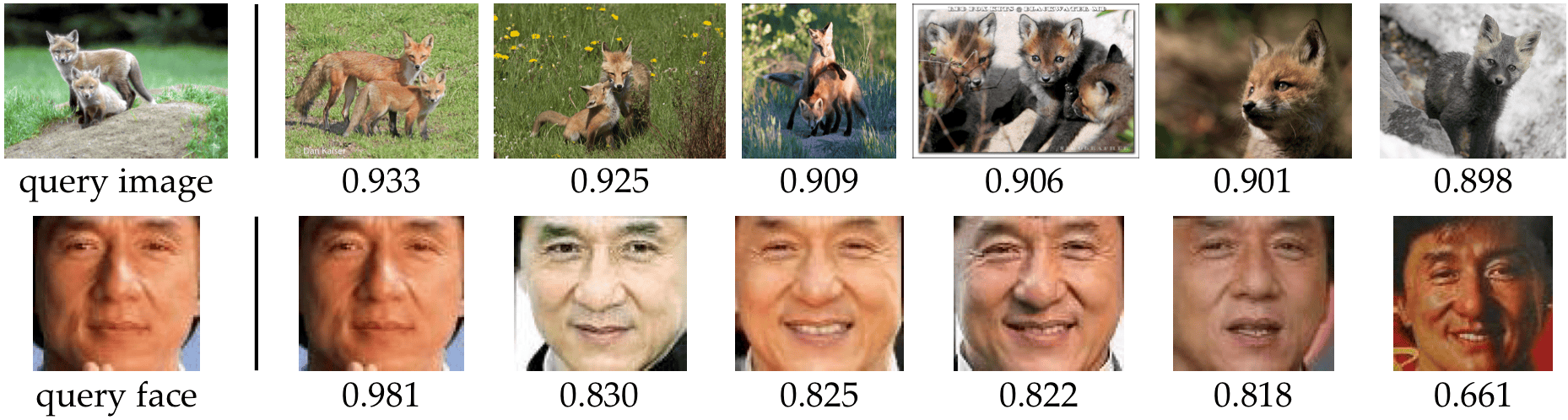• Englesma, Jain, Boddeti, "HERS: Homomorphically Encrypted Representation Search,", Arxiv 2020

## Encrypted Feature Matching (1-to-1)

Amortized Homomorphically Encrypted Inner Products• V.N. Boddeti, "Secure Face Matching Using Fully Homomorphic Encryption,", BTAS 2018

## Scalable Encrypted Search (1-to-$m$)

Image Retrieval on ImageNet (Rank-1 Accuracy in %)

Biometric Search (Rank-1 Accuracy in %)
• Englesma, Jain, Boddeti, "HERS: Homomorphically Encrypted Representation Search,", Arxiv 2020

## Two-Stage Search

• Englesma, Jain, Boddeti, "HERS: Homomorphically Encrypted Representation Search,", Arxiv 2020

## Summary

• Homomorphic encryption is a promising mechanism for privacy-preserving CV/ML.
• Doubly Permuted Homomorphic Encryption for Federated Learning: customized homomorphic encryption scheme for sparse data
• Practical Homomorphically Encrypted Image Search and Retrieval: customized data encoding schemes for 1-to-1 and 1-to-$m$ matching
Human Analysis Lab
VishnuBoddeti